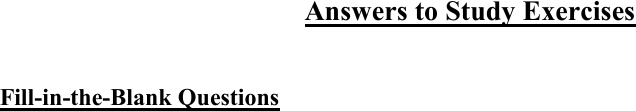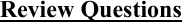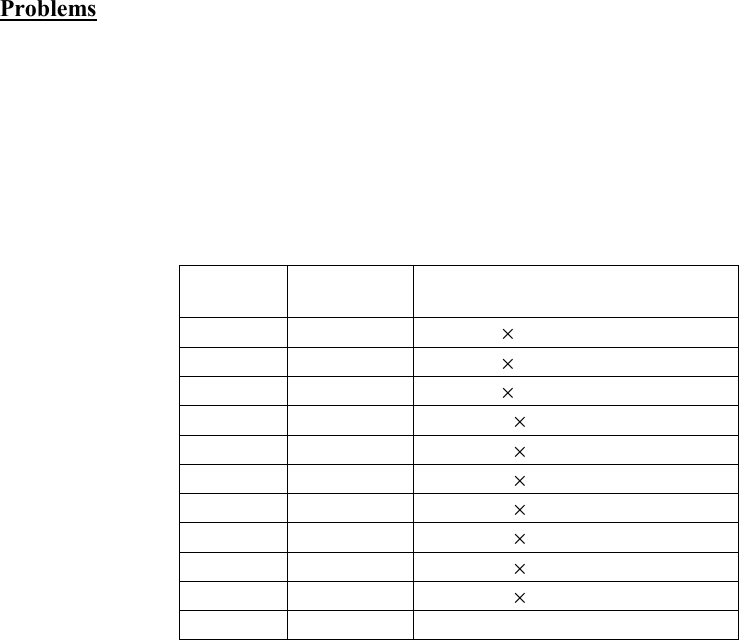Study Guides (390,000)
CA (150,000)
SFU (5,000)
BUS (800)
BUS 207 (40)
Final

# BUS 207 Chapter 2: Chapter 2Exam

Department
Course Code
BUS 207
Professor
Bernie Maroney
Study Guide
Final

This preview shows pages 1-3. to view the full 12 pages of the document.1
______________________________________________
Chapter 2: Economic Theories, Data, and Graphs
______________________________________________
Fill-in-the-Blank Questions
Question 1
a) models (or theories)
b) endogenous; exogenous
c) (conditional) prediction; empirical
d) (positively) correlated; causal
e) self-interest; utility; profits
Question 2
a) index; relative
b) absolute value of the price; absolute value of the price
c) cross-section
d) scatter
e) time-series

Only pages 1-3 are available for preview. Some parts have been intentionally blurred.2
Question 3
a) Y/X
b) 500; positively; 4
c) 12; negatively; -0.2
d) tangent
e) zero; zero
Review Questions
Question 4
a) normative (“The government should impose…” is inherently a value judgement.)
b) positive (In principle, we could determine the impact that foreign aid actually has.)
c) positive (In principle, we could determine the extent to which fee increases affect access.)
d) normative (What is or is not unfair is clearly based on a value judgement.)
e) normative (Use of the expression “too much” is a value judgement.)
Question 5
a) In the Canadian wheat sector, the amount of rainfall on the Canadian prairies is an exogenous
variable; the amount of wheat produced is an endogenous variable.
b) To the Canadian market for coffee, the world price of coffee is exogenous; the price of a cup of
coffee at Tim Horton’s is endogenous.
c) To any individual student, the widespread unavailability of student loans is exogenous; their
own attendance at university or college is endogenous.
d) To any individual driver, the tax on gasoline is exogenous; his or her own decision regarding
which vehicle to purchase is endogenous.
Question 6
The observed correlation cannot lead to a certain inference about causality. It is consistent with
the theory that the increase in demand for homes leads to an increase in the price of lumber
(which is generally a pretty sensible theory!), but it is also consistent with a different theory one
in which some unobserved factor leads to both the increase in demand for homes and separately
to the increase in the price of lumber. Correlation does not imply causality!

Only pages 1-3 are available for preview. Some parts have been intentionally blurred.3
Problems
Question 7
a) Using 2009 as the base year means that we choose \$85 as the base price. We thus divide the
actual prices in all years by \$85 and then multiply by 100. In this way, we will determine, in
percentage terms, how prices in other years differ from prices in 2009. The index values are as
follows:
Year
Price (\$)
Physics textbook price index
2009
85
(85/85) 100 = 100
2010
87
(87/85) 100 = 102.4
2011
94
(94/85) 100 = 110.6
2012
104
(104/85) 100 = 122.4
2013
110
(110/85) 100 = 129.4
2014
112
(112/85) 100 = 131.8
2015
120
(120/85) 100 = 141.2
2016
125
(125/85) 100 = 147.1
2017
127
(127/85) 100 = 149.4
2018
127
(127/85) 100 = 149.4
2019
130
(130/85) × 100 = 152.9
b) The price index in 2014 is 131.8, meaning that the price of the physics textbook is 31.8 percent
higher in 2014 than in the base year, 2009.
c) From 2016 to 2019, the price index increases from 147.1 to 152.9but this is not an increase
of 5.8 percent. The percentage increase in the price index from 2016 to 2019 is equal to [(152.9-
147.1)/147.1]×100 = 3.94 percent.
d) These are time-series data because the data are for the same product at the same place but at
different points in time.
Question 8
a) Using Calgary as the “base universitymeans that we choose \$6.25 as the base price. Thus we
divide all actual prices by \$6.25 and then multiply by 100. In this way, we will determine, in
percentage terms, how prices at other universities differ from Calgary prices. The index values
are as follows: